## Friday, July 27, 2018

### Scheduling of patients

In  a simple optimization model is presented for the scheduling of patients receiving cancer treatments.  tried to use this model. This was not so easy: small bugs in  can make life difficult when replicating things.

We use the data set in :

• There are $$T=40$$ time slots of 15 minutes
• We have 23 chairs where patients receive their treatment
• We have 8 different types of patients
• Each patient type has a demand (number of actual patients) and a treatment length (expressed in 15 minute slots)
• There is a lunch break during which no patients can start their treatment
• We want at most 2 treatment sessions starting in each time slot.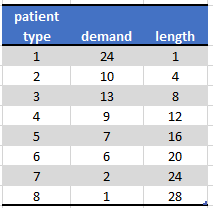Patient Data

#### Main variables

A treatment session is encoded by two binary variables: $\mathit{start}_{c,p,t} = \begin{cases} 1 & \text{if session for a patient of type p starts in time slot t in infusion chair c} \\ 0 & \text{otherwise} \end{cases}$ $\mathit{next}_{c,p,t} = \begin{cases} 1 & \text{if session for a patient of type p continues in time slot t in infusion chair c} \\ 0 & \text{otherwise} \end{cases}$Start and Next variables
Here the start variables are colored orange and the next variables are grey. Patient type 1 has a treatment session length of 1. This means a session has a start variable turned on, but no next variables.  Patient type 2 has a length of 4. So each session has one start variable and 3 next variables with values one.

Note that there are multiple patients of type 1 and 2.

#### Equations

A chair can be occupied by zero or one patients: $\sum_p \left( \mathit{start}_{c,p,t} + \mathit{next}_{c,p,t}\right)\le 1 \>\forall c,t$

When $$\mathit{start}_{c,p,t}=1$$ we need that the next $$\mathit{length}(p)-1$$ slots have $$\mathit{next}_{c,p,t'}=1$$. Here the paper  makes a mistake. They propose to model this as: $\sum_{t'=t+1}^{t+\mathit{length}(p)-1} \mathit{next}_{c,p,t'} = (\mathit{length}(p)-1)\mathit{start}_{c,p,t}\>\>\forall c,p,t$ This is not correct: this version would imply that we have $\mathit{start}_{c,p,t}=0 \Rightarrow \sum_{t'=t+1}^{t+\mathit{length}(p)-1} \mathit{next}_{c,p,t'}= 0$ This would make a lot of slots just unavailable. (Your model will most likely be infeasible). The correct constraint is:$\sum_{t'=t+1}^{t+\mathit{length}(p)-1} \mathit{next}_{c,p,t'} \ge (\mathit{length}(p)-1)\mathit{start}_{c,p,t}\>\>\forall c,p,t$ A dis-aggregated version is: $\mathit{next}_{c,p,t'} \ge \mathit{start}_{c,p,t} \>\>\forall c,p,t,t'=t+1,\dots\,t+\mathit{length}(p)-1$ This may perform a little bit better in practice (although some solvers can do such a dis-aggregation automatically).

It is noted with this formulation, we only do $\mathit{start}_{c,p,t}=1 \Rightarrow \mathit{next}_{c,p,t'}=1$ If $$\mathit{start}_{c,p,t}=0$$, we leave $$\mathit{next}_{c,p,t'}$$ unrestricted. This mean we have some $$\mathit{next}$$ variables just floating. They can be zero or one. Only the important cases are bound to be one. This again means that the final solution is just $$\mathit{start}_{c,p,t}$$, and we need to reconstruct the $$\mathit{next}$$ variables afterwards. This concept of having variables just floating in case they do not matter, can be encountered in other MIP models.

To meet demand we can do:$\sum_{c,t} \mathit{start}_{c,p,t} = \mathit{demand}_p$

Finally, lunch is easily handled by fixing $\mathit{start}_{c,p,t}=0$ when $$t$$ is part of the lunch period.

There is one additional issue: we cannot start a session if there are not enough time slots left to finish the session. I.e. we have: $\mathit{start}_{c,p,t}=0\>\>\text{if t\ge T - \mathit{length}(p)+2}$

#### GAMS model

The data looks like:

 set   c 'chair' /chair1*chair23/   p 'patient type' /patient1*patient8/   t 'time slots' /t1*t40/   lunch(t) 'lunch time' /t19*t22/ ; alias(t,tt); table patient_data(p,*)             demand  length  patient1     24     1  patient2     10     4  patient3     13     8  patient4      9    12  patient5      7    16  patient6      6    20  patient7      2    24  patient8      1    28 ; scalar maxstart 'max starts in period' /2/ ; parameter    demand(p)    length(p) ; demand(p) = patient_data(p,'demand'); length(p) = patient_data(p,'length');

We create some sets to help us make the equations simpler. This is often a good idea: sets are easier to debug than constraints. Constraints can only be verified when the whole model is finished and we can solve it. Sets can be debugged in advance. In general, I prefer constraints to be as simple as possible.

 set   startok(p,t)  'allowed slots for start'   after(p,t,tt) 'given start at (p,t), tt are further slots needed (tt = t+1..t+length-1)' ; startok(p,t) = ord(t)<=card(t)-length(p)+1; startok(p,lunch) = no; after(p,t,tt) = startok(p,t) and (ord(tt)>=ord(t)+1) and (ord(tt)<=ord(t)+length(p)-1);

The set startok looks likeSet startok
Note that lunch time slots (periods 19-22) are not available.

The set after is a bit more complicated:set after
We see here for patient type 5, given what the start period is (the start period is on the left), when the next variable needs to be turned on. E.g. when patient type 5 starts a treatment session in period 1, the next variables need to be one for periods 2 through 16. At the bottom we see again the effect of the lunch period.

The optimization model can now be expressed as:

 binary variables   start(c,p,t) 'start: begin treatment'   next(c,p,t) 'continue treatment' ; variable z 'objective variable'; start.fx(c,p,t)$(not startok(p,t)) = 0; equations obj 'dummy objective: find feasible solution only' slots(c,p,t) 'start=1 => corresponding next = 0,1' slots2(c,p,t,tt) 'disaggregated version' chair(c,t) 'occupy once' patient(p) 'demand equation' starts(t) 'limit starts in each slot' ; * dummy objective obj.. z =e= 0; * aggregated version slots(c,startok(p,t)).. sum(after(p,t,tt), next(c,p,tt)) =g= (length(p)-1) * start(c,p,t); * disaggregated version slots2(c,after(p,t,tt)).. next(c,p,tt) =g= start(c,p,t); * occupation of chair chair(c,t).. sum(p, start(c,p,t) + next(c,p,t)) =l= 1; * demand equation patient(p).. sum((c,t),start(c,p,t)) =e= demand(p); * limit starts starts(t).. sum((c,p),start(c,p,t)) =l= maxstart; model m1 /slots,chair,patient,starts,obj/; model m2 /slots2,chair,patient,starts,obj/; solve m1 minimizing z using mip; display start.l; I try to make the results more meaningful:  parameter results(*,t) 'reporting'; start.l(c,p,t) = round(start.l(c,p,t)); loop((c,p,t)$(start.l(c,p,t)=1),  results(c,t) = ord(p);  results(c,tt)after(p,t,tt) = -ord(p); ); results('starts',t) = sum((c,p),start.l(c,p,t)); We only use the start variables. We know that some of the next variables may have a value of one while not being part of the schedule. The results look like:Results The colored cells with positive numbers correspond to a start of a session. The grey cells are patients occupying a chair for the remaining time after the start slot. We see that each period has two starts, except for lunch time, when no new patients are scheduled. This model solves very quickly: about half a second. #### Proper Next variables In this model we only use the start variables for reporting. The next variables can show spurious values $$\mathit{next}_{c,p,t}=1$$ which are not binding. Can we change the model so we only have valid next variables? There are two ways: 1. Minimize the sum of next variables: $\min \sum_{c,p,t} \mathit{next}_{c,p,t}$ Surprisingly this made the model much more difficult to solve. 2. We know in advance how many next variables should be turned on. So we can add the constraint:$\sum_{c,p,t} \mathit{next}_{c,p,t} = \sum_p \mathit{demand}_p (\mathit{length}(p)-1)$ This will prevent these floating next variables. #### A better formulation We can remove the next variables altogether and use the start variables directly in the constraint that checks the occupation of chairs: $\sum_p \sum_{t'=t-\mathit{length}_p+1}^t \mathit{start}_{c,p,t'} \le 1 \> \forall c,t$ In GAMS we can model this as follows. We just need to change the set after a little bit: we let tt in after(p,t,tt) include t itself. Let's call this set cover:  set startok(p,t) 'allowed slots for start' cover(p,t,tt) 'given start at (p,t), tt are all slots needed (tt = t..t+length-1)' ; startok(p,t) = ord(t)<=card(t)-length(p)+1; startok(p,lunch) = no; cover(p,t,tt) = startok(p,t) and (ord(tt)>=ord(t)) and (ord(tt)<=ord(t)+length(p)-1); Note again that the only difference with our earlier set after(p,t,tt) is that after has (ord(tt)>=ord(t)+1) while cover(p,t,tt) has a condition: (ord(tt)>=ord(t)). One obvious difference between the sets after and cover is the handling of patient type 1. After did not have entries for this patient type, while cover shows: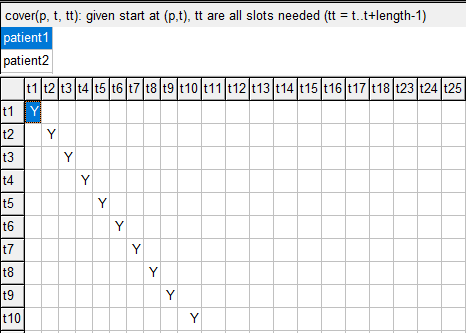Set cover has a diagonal structure for patient type 1 With this new set cover we can easily form our updated constraint chair:  * occupation of chair chair(c,t).. sum(cover(p,tt,t), start(c,p,tt)) =l= 1; This will find all variables start(c,p,tt) that potentially cover the slot (c,t). Here we see how we can simplify equations a lot by using well-designed intermediate sets. #### Minimize number of chairs We can tighten up the schedule a little bit. There is enough slack in the schedule that we actually don't need all chairs to accommodate all patients. To find the minimum number of chairs we make the following changes to the model: first we introduce a new binary variable usechair(c). Next we change the equations:  * objective obj.. z =e= sum(c, usechair(c)); * occupation of chair chair(c,t).. sum(cover(p,tt,t), start(c,p,tt)) =l= usechair(c); * chair ordering order(c-1).. usechair(c) =l= usechair(c-1); The last constraint says $$\mathit{usechair}_c \le \mathit{usechair}_{c-1}$$ for $$c\gt 1$$ (this last condition is implemented by indexing the constraint as order(c-1)). The purpose of this constraint is two-fold: (1) reduce symmetry in the model which hopefully will speed up things, and (2) make the solution better looking: all the unused chairs are at the end. With this, an optimal schedule looks like: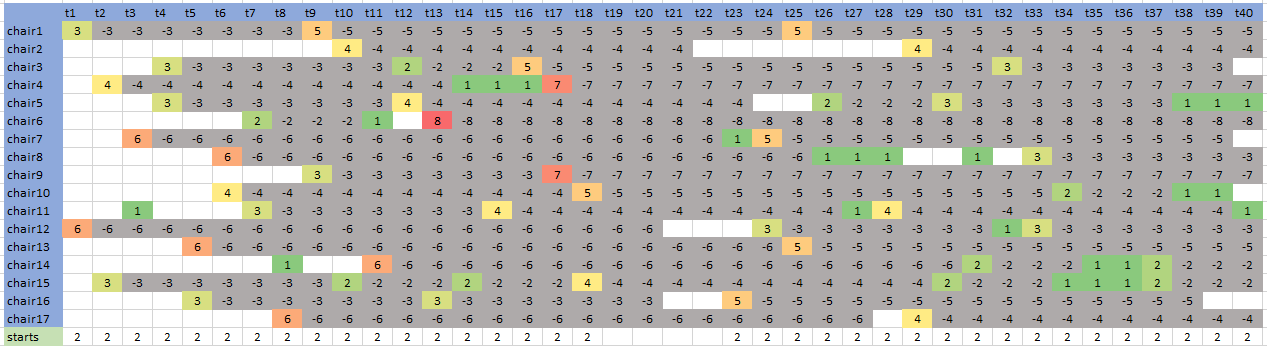Minimize number of chairs #### Multi-objective version We can try to make the schedule more compact: try to get rid of empty chairs in the middle of the schedule. An example of such a "hole" is cell: (chair6, t12). We do this by essentially solving a bi-objective problem: 1. Minimize number of chairs needed 2. Minimize spread Here the spread of a single chair is defined by last slot minus first slot the chair is used. The total spread is just the sum of these. We want to make the minimization of the number of chairs the first, most important objective. The bi-objective problem can be solved in two ways: • Solve a weighted sum objective $$w_1 z_1 + w_2 z_2$$ with a large weight on $$z_1$$ being the number of chairs used, • Solve in two steps: 1. Solve number of chairs problem 2. Fix number of chairs to optimal value and then solve minimum spread model. I used the second approach, and achieved a tighter schedule: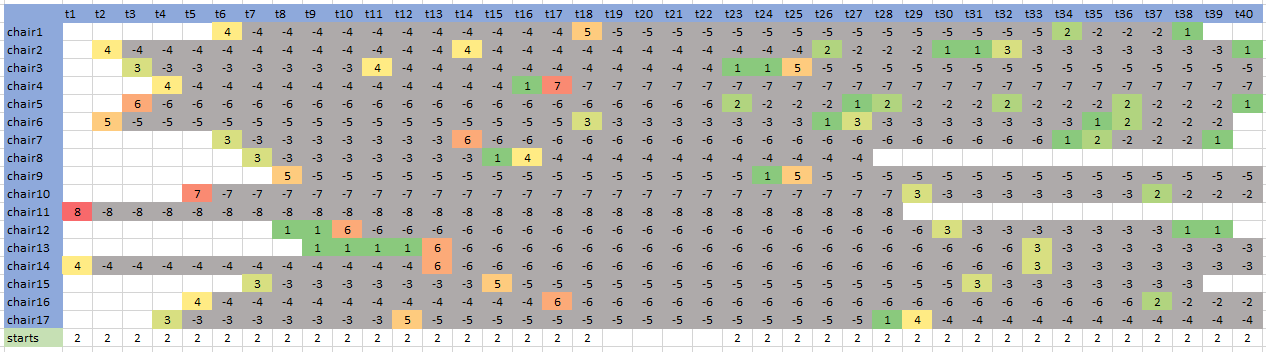Bi-objective model results Update: I added paragraphs about the suggested formulations in the comments. #### References 1. Anali Huggins, David Claudio, Eduardo Pérez, Improving Resource Utilization in a Cancer Clinic: An Optimization Model, Proceedings of the 2014 Industrial and Systems Engineering Research Conference, Y. Guan and H. Liao, eds., https://www.researchgate.net/publication/281843060_Improving_Resource_Utilization_in_a_Cancer_Clinic_An_Optimization_Model 2. Python Mixed Integer Optimization, https://stackoverflow.com/questions/51482764/python-mixed-integer-optimization ## Monday, July 23, 2018 ### Mathematical view of assignment problemExample from  I was reading some old (really old) papers on the assignment problem. The style is much more verbose than we see nowadays, and examples are very small (that is actually not a bad thing). In  (and referred to by ) is a well-known statement about mathematicians: There are, as has been indicated, a finite number of permutations in the assignment of men to jobs. When the classification problem as formulated above was presented to a mathematician, he pointed to this fact and said that from the point of view of the mathematician there was no problem. Since the number of permutations was finite, one had only to try them all and choose the best. He dismissed the problem at that point. This is rather cold comfort to the psychologist, however, when one considers that only ten men and ten jobs mean over three and a half million permutations. Trying out all the permutations may be a mathematical solution to the problem, it is not a practical solution. This is a little bit like "applied mathematics is bad mathematics"... #### References 1. Tjalling C. Koopmans and Martin Beckmann, Assignment Problems and the Location of Economic Activities, Econometrica Vol. 25, No. 1 (Jan., 1957), pp. 53-76 2. Robert L. Thorndike, The Problem of Classification of Personnel, Psychometrika, Vol. 15, No. 3, September, 1950 ## Thursday, July 19, 2018 ### Job announcement: USDA/Economic Research Service  www.usajobs.gov/GetJob/ViewDetails/505127800Baseline Data Coordinator, Economic Research Service, U.S. Department of AgricultureGrade: GS-13. (Subsequent promotion would involve a new job announcement and the standard competitive process.)Opening and closing dates for announcement on USAJOBS.gov: July 18 to July 31U.S. citizenship or naturalization would be required for employment.Description (edited -- the official description is in the link above):The incumbent will serve as an Agricultural Economist working in the area of data management, computer programming, and modeling analysis for the USDA Baseline and other scenarios. The incumbent will work directly with the Baseline Coordinators to assure effective delivery of timely, high quality products and analysis to the Office of the Secretary and other Departmental Officials. The incumbent will be responsible for providing authoritative technical analysis, and managing and manipulating large datasets covering agricultural data for multiple countries and commodities. The incumbent will be responsible for linking these datasets to models of the agricultural sectors for multiple countries to produce long-run projections for the farm sector that cover agricultural commodities, agricultural trade, and aggregate indicators for the sector. The incumbent will also be responsible for managing, updating, and writing sophisticated computer programs that are used to produce the projections.Clarification: Although the description emphasizes data work, the actual work is considerably broader. ### Graph drawing in RStudio RStudio can plot nice networks and graphs. By graph, I mean here the mathematical artifact: $$G = (V,E)$$. I.e. a collection of nodes and arcs. DiagrammeR is an R package that includes graphviz. RStudio has some support for graphviz input files (editing, drawing). Basically we need to specify nodes and arcs, and let the tool do its work.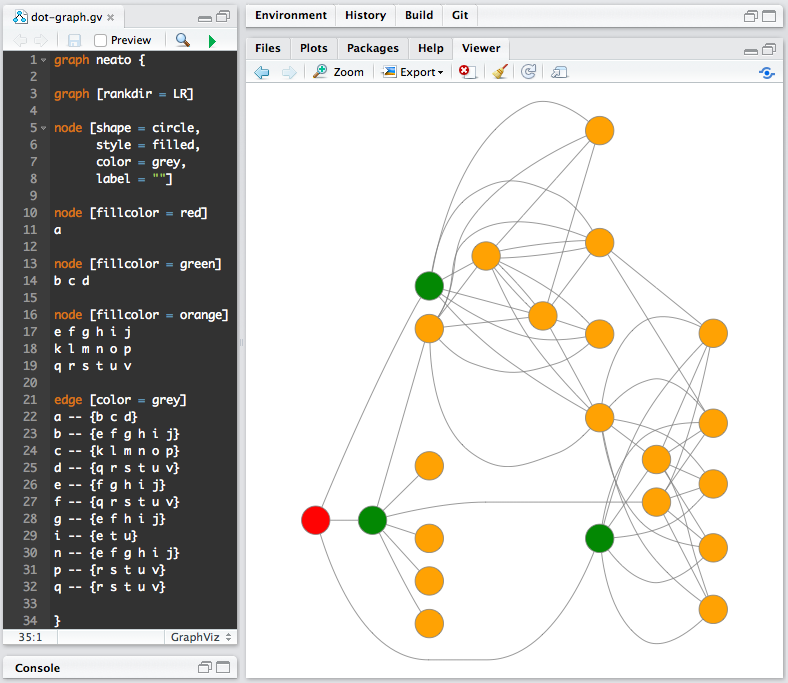Graphviz file in RStudio (picture from )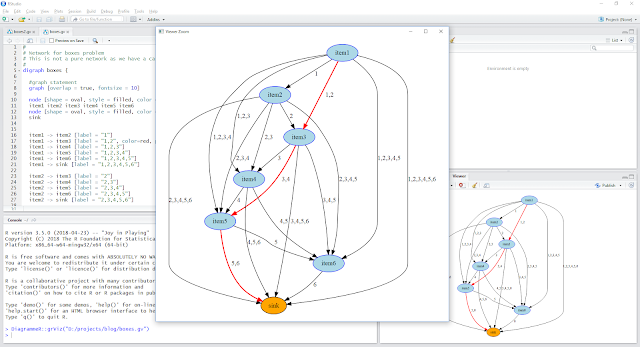Network model used in  I like it. #### References 1. DiagrammeR Docs, graphviz, http://rich-iannone.github.io/DiagrammeR/graphviz_and_mermaid.html 2. Graphviz, Node, Edge and Graph attributes, https://graphviz.gitlab.io/_pages/doc/info/attrs.html 3. Choosing boxes: set covering vs network models vs dynamic programming, http://yetanothermathprogrammingconsultant.blogspot.com/2018/07/choosing-boxes-set-covering-model.html ## Saturday, July 14, 2018 ### Choosing boxes: set covering vs network models vs dynamic programmingFrom : I have the following problem: 1. I have N square paper documents with side lengths between 150mm and 860mm. I know each document side's length. 2. I need to create 3−4 differently sized boxes to fit all the documents, e.g. Three box types: Box 1 side L1=300mm, Box 2 side L2=600mm, Box 3: L3=860mm. 3. There are as many boxes as documents, i.e. each document goes into its own separate box (of the smallest possible size so as to minimize waste of cardboard). 4. What is the best way to decide on the size of the boxes, so as to minimize the total amount of (surface area) of cardboard used? The data looks like:Original Data (partial view) We have 1166 records. We see there are items with the same length. These duplicates will always go in the same box type. So we don't have to distinguish them in the optimization model. As a result, we can reorganize the data into unique values and their count: --- 392 PARAMETER data size count i1 156.000 1.000 i2 162.000 1.000 i3 168.000 1.000 i4 178.000 2.000 i5 180.000 1.000 i6 185.000 2.000 ... i379 806.000 1.000 i380 820.000 1.000 i381 823.000 1.000 i382 827.000 2.000 i383 855.000 2.000 i384 864.000 1.000  This table has 384 records. A big improvement over 1166 items! This will make our optimization models much smaller. Note that we make sure things are ordered by size (increasing). We will exploit this in one of the models below. #### Small data example For small data sets we can easily enumerate all possible ways to use $$T$$ different box sizes. Here we have $$n=6$$ unique item sizes and $$T=3$$ boxes: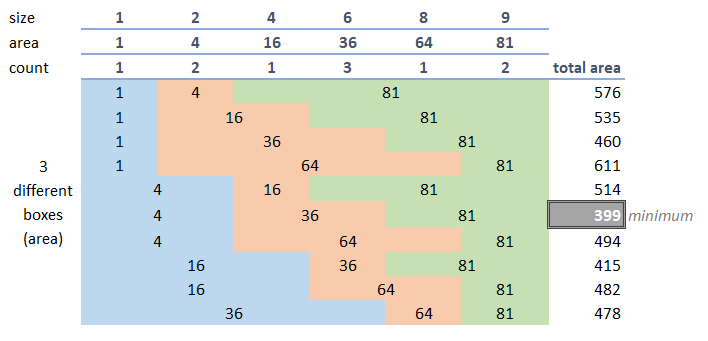Small data set with n=6, T=3 We see that the best configuration is to have boxes of size 2, 6 and 9 with areas 4. 36 and 81. We assume throughout that each box is used for at least one item. This makes sense: when adding a new box size, it is never a good idea not to use it. Remember: box sizes are variable: we determine the size of a box (it is not given). Some more observations: • The larges box size is the size of the largest item. • It will never make sense to choose a box size that is not equal to one of the item sizes. In this example: a box size of 3 will never be optimal. For large problems, of course, complete enumeration is out of the question. We will need to do something more cleverly. #### Assignment formulation It is tempting to start with an assignment variable such as: $x_{i,j} = \begin{cases} 1 & \text{if item i is assigned to box type j=1,\dots\,T}\\ 0 & \text{otherwise}\end{cases}$ Here $$T$$ is the number of differently sized boxes. Furthermore let $a_j = \text{area of box j}$ This can lead to a model like: \bbox[lightcyan,10px,border:3px solid darkblue] {\begin{align}\min & \sum_{i,j} \> \mathit{count}_i \> a_j \> x_{i,j} \\ & \sum_j x_{i,j} = 1 & \forall i & \> \> \text{(assignment)} \\ & x_{i,j} = 1 \Rightarrow a_j \ge \mathit{size}_i^2 & &\>\>\text{(fit)} \\ & x_{i,j} \in \{0,1\} \\ & a_j \ge 0 && \>\>\text{(area of box type j)}\end{align}} This is problematic: we have a non-convex quadratic objective. This model can be linearized with some effort. First we introduce a variable $$y_{i,j}=a_j x_{i,j}$$. As we are minimizing, we can get away with just $$y_{i,j} \ge a_j - M (1-x_{i,j})$$. The linearized model can look like \bbox[lightcyan,10px,border:3px solid darkblue] {\begin{align}min & \sum_{i,j} \> \mathit{count}_i \> y_{i,j} \\ & a_j \ge \mathit{size}_i^2 \> x_{i,j} \\ & y_{i,j} \ge a_j - \mathit{Amax} (1-x_{i,j}) \\ & a_1 = \mathit{Amax} \\ & a_{j+1} \le a_j - 1 \end{align}} where $$\mathit{Amax}=864^2$$. The last two constraints should help the solver a little bit: we reduce symmetry. This model is very difficult to solve. After 20 minutes it still had a gap of 30%. #### Covering formulation This model is organized around the variable $x_{i,j} = \begin{cases} 1 & \text{if items i through j are put in the same size box (with size j)} \\ 0 & \text{otherwise}\end{cases}$ This is somewhat unusual, but we'll see this will make a rather nice MIP model. Here we also see why the ordering of items by size is a useful concept: if $$x_{i,j}=1$$ then all items $$i,i+1,\dots,j-1,j$$ are placed in the same size box. The basic idea is to cover each item exactly once by a variable $$x_{i,j}$$. In addition we want exactly $$T$$ variables that have a value of one. Here is how we organize our variables $$x_{i,j}$$: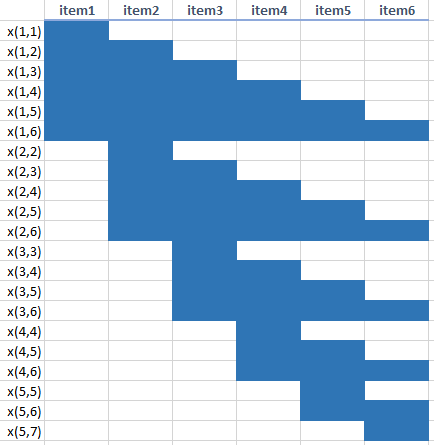Variables x(i,j) A feasible solution has two properties: • Each item (column) selects exactly one variable (row), • The total number of selected variables is $$T$$. A feasible solution can look like:Feasible solution for T=3 The feasible solution has exactly $$T=3$$ variables selected, and all items are covered exactly once. The complete model is surprisingly simple: \bbox[lightcyan,10px,border:3px solid darkblue] {\begin{align}min & \sum_{i\le j} c_{i,j} x_{i,j}\\ & \sum_{i \le k \le j} x_{i,j} = 1 & \forall k\\ & \sum_{i\le j} x_{i,j} = T \\ & x_{i,j} \in \{0,1\}\end{align}} The constraint $\sum_{i \le k \le j} x_{i,j} = 1\>\>\> \forall k$ is typically a long summation. In the picture above, for $$k=2$$, we have to add up the variables $$x_{1,2}$$, $$x_{1,3}$$, $$x_{1,4}$$, $$x_{1,5}$$, $$x_{1,6}$$, $$x_{2,2}$$, $$x_{2,3}$$, $$x_{2,4}$$, $$x_{2,5}$$, and $$x_{2,6}$$. The cost coefficients can be calculated as: $c_{i,j} = \sum_{k=i}^j \mathit{count}_k \>\mathit{size}_j^2 \>\>\>\>\forall i\le j$ or if you prefer $c_{i,j} = \mathit{size}_j^2 \sum_{i\le k\le j} \mathit{count}_k \ \>\>\>\>\forall i\le j$ This is like a set covering or better set partitioning problem (we want to cover exactly once: this is a set partitioning construct). This leads to a large, but easy to solve MIP model: for $$T=4$$, I see: MODEL STATISTICS BLOCKS OF EQUATIONS 3 SINGLE EQUATIONS 386 BLOCKS OF VARIABLES 2 SINGLE VARIABLES 73,921 NON ZERO ELEMENTS 9,658,881 DISCRETE VARIABLES 73,920  This looks scary, but actually solves very fast: Root relaxation solution time = 6.73 sec. (3375.50 ticks) Nodes Cuts/ Node Left Objective IInf Best Integer Best Bound ItCnt Gap * 0+ 0 7.96958e+08 0.0000 100.00% Found incumbent of value 7.9695838e+08 after 102.45 sec. (46615.58 ticks) * 0 0 integral 0 3.29199e+08 3.29199e+08 2111 0.00% Elapsed time = 102.56 sec. (46669.97 ticks, tree = 0.00 MB, solutions = 2) Found incumbent of value 3.2919912e+08 after 102.56 sec. (46669.97 ticks)  The results look like: ---- 447 PARAMETER results count minsize maxsize area sum area i1 .i155 549.000 156.000 388.000 150544.000 8.264866E+7 i156 .i268 377.000 389.000 550.000 302500.000 1.140425E+8 i269 .i351 187.000 552.000 705.000 497025.000 9.294368E+7 i352 .i384 53.000 710.000 864.000 746496.000 3.956429E+7 total. 1166.000 3.291991E+8  This means we put items 1 through 155 in the smallest box with size 388 (area = $$388^2$$), etc. When we run the model with $$T=3$$ we see: ---- 447 PARAMETER results count minsize maxsize area sum area i1 .i155 549.000 156.000 388.000 150544.000 8.264866E+7 i156 .i284 423.000 389.000 574.000 329476.000 1.393683E+8 i285 .i384 194.000 576.000 864.000 746496.000 1.448202E+8 total. 1166.000 3.668372E+8  Indeed the total cost (area) goes up. Interestingly the smallest box size stays the same. #### GAMS implementation  set i /i1*i384/; alias(i,j,k); set ij(i,j) 'allowed i,j combinations: i<=j' ijk(i,j,k) 'allowed i,j,k combinations: i<=k<=j' ; ij(i,j) = ord(i)<=ord(j); ijk(ij(i,j),k) = ord(k)>=ord(i) and ord(k)<=ord(j); table data(i,*) size count i1 156 1 i2 162 1 i3 168 1 i4 178 2 . . . i381 823 1 i382 827 2 i383 855 2 i384 864 1 ; parameter count(i), size(i); count(i) = data(i,'count'); size(i) = data(i,'size'); scalar T 'number of different box sizes' /4/; binary variables x(i,j) 'items i-j in a single box type'; variable totalarea; parameter c(i,j) 'cost when items i-j are in box with size size(j)'; c(ij(i,j)) = sum(ijk(ij,k), count(k)*sqr(size(j))); equations cover(k) 'cover each item k exactly once' numboxes 'T different box sizes' objective 'minimize total area' ; cover(k).. sum(ijk(ij,k),x(ij)) =e= 1; numboxes.. sum(ij,x(ij)) =e= T; objective.. totalarea =e= sum(ij, c(ij)*x(ij)); model m /all/; option optcr=0; solve m minimizing totalarea using mip; set xij(i,j) 'selected x(i,j)'; xij(i,j) = x.l(i,j) > 0.5; parameter results(*,*,*);results(xij(i,j),'minsize') = size(i); results(xij(i,j),'maxsize') = size(j); results(xij(i,j),'area') = sqr(size(j)); results(xij,'count') = sum(ijk(xij,k),count(k)); results(xij,'sum area') = c(xij); results('total','','sum area') = totalarea.l; results('total','','count') = sum(ijk(xij,k),count(k)); display results; Notes: • Intermediate sets are used to simplify things. I use this approach a lot. Sets are easier to debug than equations. • Reporting is important. I try to collect meaningful information in the 3d parameter results. Hopefully the results can be interpreted even when not knowing the model or even when not knowing GAMS. • The set xij(i,j) will indicate where we have x.l(i,j)=1. In practice, values for a binary variable can be 0.99999 or 1.00001. So I don't test against 1.0 exactly. • I left out the data step where I combine duplicate item sizes. That was done in a separate piece of GAMS code. #### Network model I This problem can also be modeled as a network problem. See  for more details. The network is not very simple. Consider again the 6 item, $$T=3$$ example. We drew a picture of a feasible covering earlier. The same feasible solution for the network of this problem looks like: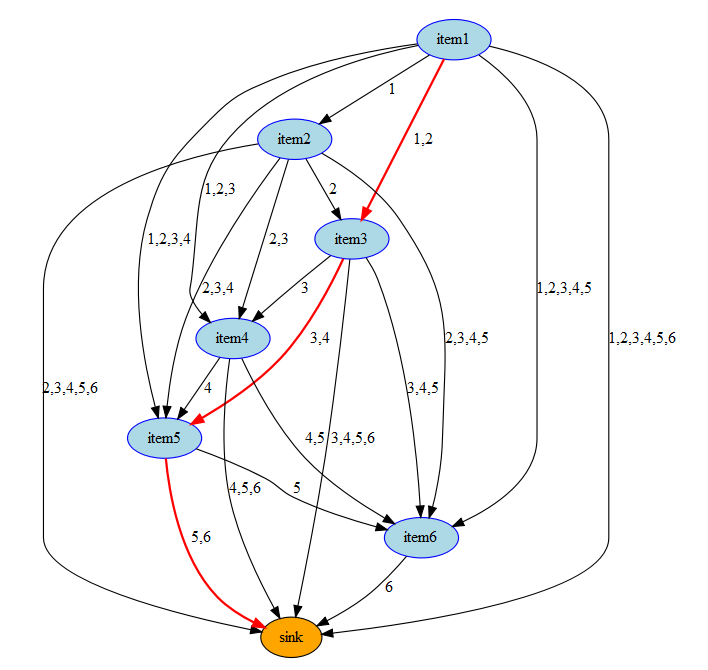Feasible solution for T=3 Selecting a link from item $$i$$ to item $$j$$ means: put items $$i,i+1,\dots,j-1$$ into a box with size $$j-1$$. Similarly, a link from item $$i$$ to the sink node means: put items $$i,i+1,\dots,n$$ into a box with size $$n$$ (where $$n$$ is the largest item or box). The cost coefficients are defined by: \begin{align}c_{i,j} &= \mathit{size}_{j-1}^2 \sum_{k=i}^{j-1} \mathit{count}_k & \forall i\le j\\c_{i,\mathit{sink}} &= \mathit{size}_n^2 \sum_{k=i}^n \mathit{count}_k & \forall i \end{align} We want to solve a shortest path problem from item 1 to the sink node with a side constraint: the number of "blue" nodes we visit should be exactly $$T$$. The side constraint makes this a cardinality constrained or hop constrained shortest path problem. We can solve this as an MIP problem: \bbox[lightcyan,10px,border:3px solid darkblue] {\begin{align}min & \sum_{(i,j)\in A} c_{i,j} x_{i,j} \\ & \sum_{(j,i)\in A} x_{j,i} + b_i = y_i & \forall i & \>\> \text{(flow balance)}\\ & y_i = \sum_{(i,j)\in A} x_{i,j} & \forall i & \>\>\text{(outflow node i)}\\ &\sum_{i \ne \mathit{sink}} y_i = T &&\text{(cardinality)}\\ & x_{i,j},y_i \ge 0 \end{align} } Here $$b_i$$ is the net exogenous supply of node $$i$$. I.e. $b_i = \begin{cases} 1 & \>\> \text{if i=1}\\ -1 & \>\> \text{if i=\mathit{sink}}\\ 0 & \>\>\text{otherwise}\end{cases}$ This model solves very fast (the relaxed LP gives an integer solution for this data set). The solution for our real data with $$T=4$$ looks like: ---- 470 VARIABLE cost.L = 3.291991E+8 ---- 470 VARIABLE x.L arcs i156 i269 i352 sink i1 1.000 i156 1.000 i269 1.000 i352 1.000  This means our optimal grouping is $$i_1 - i_{155}$$, $$i_{156} - i_{268}$$, $$i_{269} - i_{351}$$, $$i_{352} - i_{384}$$. This is the same solution as for our set partitioning model. #### GAMS Code The GAMS code for this model can look like: ontext    Select Boxes    Cardinality constrained shortest path $offtext sets iext 'orig nodes + sink' /i1*i384,sink/ i(iext) 'orig nodes w/o sink' /i1*i384/ ; table data(i,*) size count i1 156 1 i2 162 1 i3 168 1 i4 178 2 . . . i381 823 1 i382 827 2 i383 855 2 i384 864 1 ; parameter count(i), size(i); count(i) = data(i,'count'); size(i) = data(i,'size'); scalar T 'number of different box sizes' /4/; alias(iext,jext); alias(i,j,k); sets ij(i,j) 'allowed i,j combinations: i<=j' ijk(i,j,k) 'allowed i,j,k combinations: i<=k<=j' ; singleton set lastj(j) 'last element of j'; ij(i,j) = ord(i)<=ord(j); ijk(ij(i,j),k) = ord(k)>=ord(i) and ord(k)<=ord(j); lastj(j) = ord(j)=card(j); * * network topology * set a(iext,iext) 'arcs'; a(ij) = yes; a(i,'sink') = yes; parameter c(iext,iext) 'cost coefficients'; c(a(i,j)) = sqr(size(j-1)) * sum(ijk(i,j-1,k),count(k)); c(i,'sink') = sqr(size(lastj)) * sum(ijk(i,lastj,k),count(k)); parameter pinflow(iext) 'source/sink' / sink -1 i1 1 /; binary variables x(iext,iext) 'arcs' y(iext) 'outflow of node' ; variables cost; equations nodbal 'node balance' calcoutflow 'calc y' numboxes 'we need an active node for box' obj 'objective' ; obj.. cost =e= sum(a,c(a)*x(a)); calcoutflow(iext).. y(iext) =e= sum(a(iext,jext),x(a)); nodbal(iext).. sum(a(jext,iext), x(a)) + pinflow(iext) =e= y(iext); numboxes.. sum(i,y(i)) =e= T; model m /all/; * for this data set we can solve as LP (to be safe solve as MIP) solve m minimizing cost using rmip; display cost.l, x.l; * check if close to integer solution abort$sum((iext,jext)$(abs[x.l(iext,jext)-round(x.l(iext,jext))]>1e-4),1) "non-integer solution: solve as MIP"; * * reporting * set xij(i,jext) 'items i..j to into same box'; xij(i,jext) = x.l(i,jext+1) > 0.5; parameter results(*,*,*); results(xij(i,j),'minsize') = size(i); results(xij(i,j),'maxsize') = size(j); results(xij(i,j),'area') = sqr(size(j)); results(xij(i,j),'count') = sum(ijk(i,j,k),count(k)); results(xij(i,j),'sum area') = sqr(size(j)) * sum(ijk(i,j,k),count(k)); results('total','','sum area') = cost.l; results('total','','count') = sum(ijk(xij(i,j),k),count(k)); display results; #### Network Model II The previous model was a network model with a side constraint. We can create a pure network by augmenting the network. Basically we keep track of the number of different boxes by indexing the nodes by item number and layer number. This means we have a larger number of nodes. The network can look like:Layered network Here we see all paths contain $$T=3$$ blue nodes. Each node is labeled by (item,layer). Be aware that an arc $$i \rightarrow j$$ represents putting items $$i,\dots,j-1$$ in the same sized box. As nodes have 2 indices, arcs have four! The results for the original data set with $$T=4$$ looks like: ---- 467 VARIABLE cost.L = 3.291991E+8 ---- 467 VARIABLE x.L arcs i156.layer2 i269.layer3 i352.layer4 sink.Lsink i1 .layer1 1.00 i156.layer2 1.00 i269.layer3 1.00 i352.layer4 1.00  Note again that this must be interpreted as follows: group items $$i_1 - i_{155}$$, $$i_{156} - i_{268}$$, $$i_{269} - i_{351}$$, $$i_{352} - i_{384}$$ in box sizes large enough to hold $$i_{155}$$, $$i_{268}$$, $$i_{351}$$, $$i_{384}$$ . This is the same solution as for our earlier models. #### GAMS Model $ontext    Select Boxes    Shortest path in layered network \$offtext sets  iext 'orig nodes + sink' /i1*i384,sink/  i(iext) 'orig nodes w/o sink' /i1*i384/  Lext 'orig layers + sink' /layer1*layer4,Lsink/  L(Lext) 'lyaers w/o sink' /layer1*layer4/ ; table data(i,*)            size      count i1          156        1 i2          162        1 i3          168        1 i4          178        2 . . . i381        823        1 i382        827        2 i383        855        2 i384        864        1 ; parameter count(i), size(i); count(i) = data(i,'count'); size(i) = data(i,'size'); scalar T 'number of different box sizes'; T = card(L); alias(iext,jext); alias(i,j,k); alias(Lext,L1ext,L2ext); alias(L,L1,L2); sets   ij(i,j)      'allowed i,j combinations: i<=j'   ijk(i,j,k)   'allowed i,j,k combinations: i<=k<=j' ; singleton sets   lastj(j)     'last element of j'   lastL(L)     'last element of L' ; ij(i,j) = ord(i)<=ord(j); ijk(ij(i,j),k) = ord(k)>=ord(i) and ord(k)<=ord(j); lastj(j) = ord(j)=card(j); lastL(L) = ord(L)=card(L); * * network topology * set a(iext,L1ext,jext,L2ext) 'arcs'; a(i,L1,j,L1+1) = ord(j)>ord(i); a(i,lastL,'sink','Lsink') = yes; parameter c(iext,L1ext,jext,L2ext) 'cost coefficients'; c(a(i,L1,j,L2)) = sqr(size(j-1)) * sum(ijk(i,j-1,k),count(k)); c(i,lastL,'sink','Lsink') = sqr(size(lastj)) * sum(ijk(i,lastj,k),count(k)); parameter pinflow(iext,Lext)  'source/sink' /    sink.Lsink   -1    i1.layer1     1 /; binary variables   x(iext,Lext,iext,Lext)  'arcs' ; variables cost; equations   nodbal       'node balance'   obj          'objective' ; obj.. cost =e= sum(a,c(a)*x(a)); nodbal(iext,L1ext)..   sum(a(jext,L2ext,iext,L1ext), x(a)) + pinflow(iext,L1ext) =e= sum(a(iext,L1ext,jext,L2ext), x(a)); model m /all/; * this is a pure network: we can solve as an LP solve m minimizing cost using rmip; option x:2:2:2; display cost.l, x.l; * * reporting * set xij(i,jext) 'items i..j to into same box'; xij(i,jext) = sum((L1ext,L2ext),x.l(i,L1ext,jext+1,L2ext)) > 0.5; parameter results(*,*,*); results(xij(i,j),'minsize') = size(i); results(xij(i,j),'maxsize') = size(j); results(xij(i,j),'area') = sqr(size(j)); results(xij(i,j),'count') = sum(ijk(i,j,k),count(k)); results(xij(i,j),'sum area') = sqr(size(j)) * sum(ijk(i,j,k),count(k)); results('total','','sum area') = cost.l; results('total','','count') = sum(ijk(xij(i,j),k),count(k)); display results;

As this is a pure network, we can ask Cplex to use the network simplex method to solve this. We see:

---   1,537 rows  220,993 columns  662,977 non-zeroes
---   220,992 discrete-columns
--- Executing CPLEX: elapsed 0:01:15.411

...

Tried aggregator 1 time.
LP Presolve eliminated 393 rows and 219470 columns.
Aggregator did 764 substitutions.
Reduced LP has 380 rows, 759 columns, and 1138 nonzeros.
Presolve time = 2.25 sec. (568.28 ticks)
Extracted network with 381 nodes and 759 arcs.
Extraction time = 0.00 sec. (0.11 ticks)

Iteration log . . .
Iteration:     0   Infeasibility     =             1.000000 (24337)
Iteration:   100   Infeasibility     =             1.000000 (24337)
Iteration:   200   Infeasibility     =             1.000000 (24337)

Network - Optimal:  Objective =    3.2919911900e+08
Network time = 0.00 sec. (0.07 ticks)  Iterations = 261 (261)
LP status(1): optimal
Cplex Time: 2.39sec (det. 588.24 ticks)


We see the presolver takes most of the time, but is also highly effective. The presolved model takes zero seconds to solve.

#### Dynamic Programming

A dynamic programming algorithm is an alternative for this problem. If we define $f_{i,b} = \text{minimum total area when we pack items 1,\dots,i (ordered and with unique size) in b box sizes}$ then we can write down the recursion:$f_{i,b} = \min_{k=b-1,\dots,i-1} \left\{ f_{k,b-1} + \mathit{size}_i^2 \sum_{j=k+1}^i \mathit{count}_j \right\}$

When we code this up in R we could see something like:

data <- c(
156,162,168,178,178,180,185,185,190,192,193,194,195,195,197,197,198,198,199,200,202,206,
206,210,210,210,212,212,214,215,217,217,217,217,217,218,220,220,220,220,220,220,220,220,
...
727,730,733,733,734,734,734,735,735,735,738,740,743,744,754,755,755,755,755,755,758,760,
766,766,780,780,780,780,780,780,780,782,783,785,795,805,806,820,823,827,827,855,855,864)

t<-table(data)
count <- as.vector(t)
size <- as.numeric(rownames(t))

cumulative <- cumsum(count)

# number of box sizes, number of item sizes
NB <- 4
NI <- length(size)

# allocate matrix NI rows, NB columns (initialize with NAs)
# f[ni,nb] = cost when we have ni items and nb blocks
F <- matrix(NA,NI,NB)
S <- matrix("",NI,NB)

# initialize for nb=1
F[1:NI,1] <- cumulative * size[1:NI]^2
S[1:NI,1] <- paste("(",1,"-",1:NI,")",sep="")

# dyn programming loop
for (nb in 2:NB) {
for (ni in nb:NI) {
k <- (nb-1):(ni-1)
v <- F[k,nb-1] + (cumulative[ni]-cumulative[k])*size[ni]^2
F[ni,nb] <- min(v)

# create path (string)
mink <- which.min(v) + nb - 2
s <- paste("(",mink+1,"-",ni,")",sep="")
S[ni,nb] <- paste(S[mink,nb-1],s,sep=",")
}
}

for (nb in 1:NB) {
cat(sprintf("%s boxes: %s, total area = %g\n",nb,S[NI,nb],F[NI,nb]))
}


Note that the inner $$k$$ loop is vectorized. The results look like:

1 boxes: (1-384), total area = 8.70414e+08
2 boxes: (1-268),(269-384), total area = 4.59274e+08
3 boxes: (1-155),(156-284),(285-384), total area = 3.66837e+08
4 boxes: (1-155),(156-268),(269-351),(352-384), total area = 3.29199e+08


#### Data

The data set used here is shown below:

  156,162,168,178,178,180,185,185,190,192,193,194,195,195,197,197,198,198,199,200,202,206
206,210,210,210,212,212,214,215,217,217,217,217,217,218,220,220,220,220,220,220,220,220
220,220,222,223,223,224,225,225,225,225,225,225,225,226,226,226,227,228,228,228,228,230
230,230,230,230,230,230,230,230,230,230,230,230,232,232,232,233,233,234,234,235,235,235
235,238,238,238,238,240,240,240,240,240,240,240,240,240,241,242,242,242,242,243,244,244
244,245,246,247,247,247,249,250,250,250,250,250,251,252,252,252,252,253,254,254,254,255
255,255,255,255,256,256,257,257,257,258,258,258,258,258,259,260,260,260,260,260,260,260
260,260,262,262,262,262,262,262,262,264,264,264,265,265,265,265,265,266,267,267,267,267
268,268,268,268,268,268,269,270,270,270,270,270,270,270,270,270,270,271,272,272,272,272
272,273,273,273,273,274,274,274,274,274,275,275,275,275,275,275,277,277,277,278,278,278
278,278,278,280,280,280,280,281,282,282,284,285,285,285,285,285,285,287,287,287,288,288
288,288,288,289,290,290,290,290,290,290,290,290,290,290,290,292,292,293,293,294,294,294
295,295,295,295,295,295,295,295,296,296,297,297,298,298,298,298,300,300,300,300,300,300
300,300,300,300,300,300,300,300,302,302,303,303,303,303,304,305,305,305,305,305,306,306
307,308,308,308,310,310,310,310,310,310,310,312,312,312,312,313,315,315,315,315,315,315
315,315,315,317,317,317,317,318,318,318,318,320,320,320,320,320,320,320,320,320,320,320
320,320,322,323,323,325,325,325,325,326,326,327,327,328,328,330,330,330,330,330,330,330
332,333,333,334,334,334,334,335,335,335,335,335,336,336,336,338,338,339,339,340,340,340
340,340,340,340,342,342,342,342,343,345,345,345,345,345,345,345,346,346,346,347,347,348
348,348,349,350,350,350,350,350,350,350,350,350,350,350,350,350,350,350,350,350,350,352
352,353,353,353,353,354,354,354,356,357,357,357,357,358,358,360,360,360,360,360,360,360
360,360,360,360,360,361,362,362,362,363,363,364,364,364,364,365,365,365,365,365,365,365
367,367,367,368,370,370,370,370,370,370,370,370,370,372,372,372,373,375,375,375,375,375
375,377,377,377,378,380,380,380,380,380,380,380,380,380,380,380,380,381,382,383,383,383
383,384,384,384,384,385,385,385,386,386,386,386,387,387,387,387,388,388,388,388,388,389
390,392,392,393,394,394,395,396,397,397,398,398,398,400,400,400,400,400,400,400,400,405
405,408,410,410,410,410,410,410,412,412,412,412,412,413,415,415,416,416,417,417,418,418
419,419,419,420,420,420,420,420,420,420,420,423,423,423,423,424,424,425,425,425,428,428
430,430,430,430,430,430,430,430,430,430,430,430,431,432,432,432,432,433,433,433,433,433
434,434,435,435,435,435,437,438,438,438,438,440,440,440,440,440,440,440,441,442,443,444
444,445,445,445,445,445,445,445,445,446,447,448,448,450,450,450,450,450,450,450,450,450
452,453,453,455,455,455,455,456,457,458,458,458,458,459,460,460,460,460,460,460,460,462
462,463,464,465,465,465,465,465,465,465,466,466,467,467,467,467,467,468,468,468,469,469
470,470,470,470,470,470,470,470,470,472,472,472,474,475,475,476,478,478,478,478,480,480
480,480,480,480,480,480,480,480,480,480,482,482,482,482,482,482,482,485,485,485,485,488
488,488,488,490,490,490,490,490,490,490,490,491,491,492,492,492,492,492,492,492,493,493
495,495,495,496,497,498,498,498,498,498,498,498,499,500,500,500,500,500,500,502,502,502
505,505,505,505,505,506,507,507,510,510,510,510,510,510,510,510,510,510,510,510,512,512
513,514,515,515,517,517,518,520,520,520,520,520,520,522,523,523,523,524,525,525,525,525
525,525,528,528,528,528,528,528,528,530,530,530,530,530,530,531,532,534,535,535,535,535
535,536,538,538,538,539,540,540,540,540,540,540,540,540,540,540,540,541,543,543,543,544
544,545,545,545,546,546,546,547,547,548,548,550,550,550,550,550,550,550,550,550,550,550
550,550,552,552,555,555,555,555,555,555,555,557,557,557,557,560,560,560,560,560,562,562
563,563,564,565,565,565,565,565,565,567,567,568,568,569,569,569,570,570,570,571,572,572
573,574,574,574,576,576,578,580,580,580,584,585,585,587,588,590,592,593,596,597,600,600
600,600,602,602,602,602,602,603,604,605,605,605,605,605,605,605,607,607,607,608,610,610
610,610,610,610,612,612,612,612,615,615,615,615,618,618,620,622,624,625,625,625,627,627
628,628,628,630,630,632,633,635,635,637,638,638,640,640,640,640,640,642,643,647,648,649
650,650,655,655,655,656,660,660,660,660,660,662,662,663,664,664,664,664,665,665,665,670
670,672,677,677,679,680,680,680,680,680,685,685,687,688,690,690,692,694,695,697,698,700
700,701,704,704,705,705,705,705,705,705,705,705,705,710,710,712,715,717,720,720,722,723
727,730,733,733,734,734,734,735,735,735,738,740,743,744,754,755,755,755,755,755,758,760
766,766,780,780,780,780,780,780,780,782,783,785,795,805,806,820,823,827,827,855,855,864


#### References

1. Need to create 3−4 different box sizes and to minimize material waste for a set of n objects that need to fit into these boxes, https://math.stackexchange.com/questions/2843990/need-to-create-3-4-different-box-sizes-and-to-minimize-material-waste-for-a-se/2850260